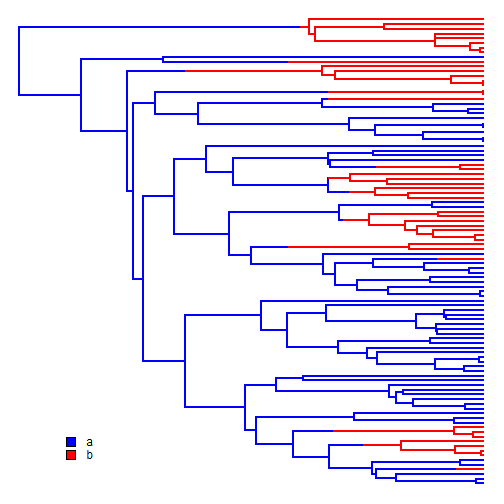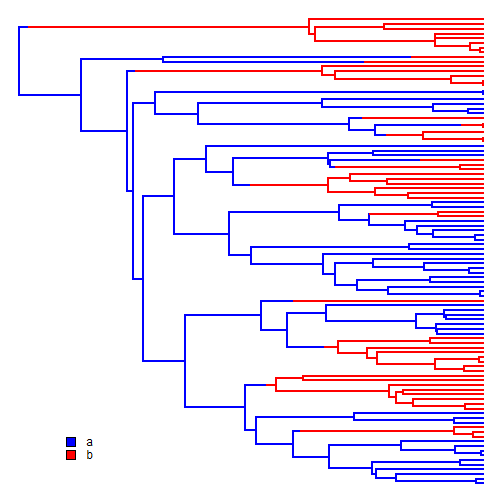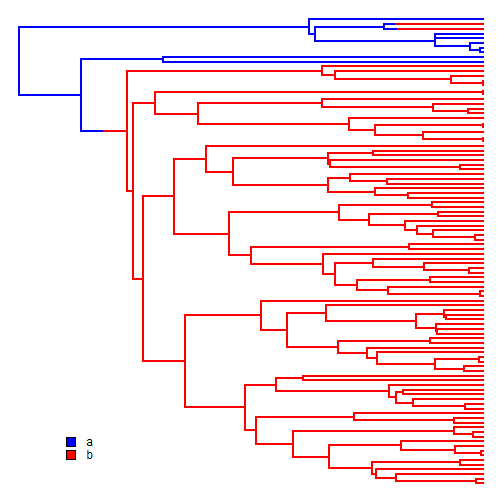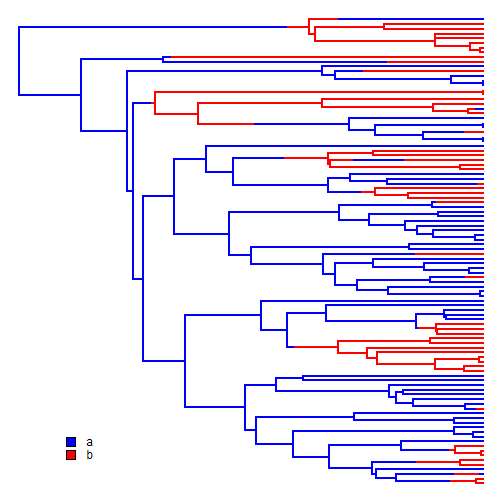## Thursday, September 18, 2014

### Update to sim.history including sensible error handling

For a long time, the phytools function `sim.history`, which simulates a stochastic character history under a model, has (backwardly, I'm afraid to say) taken as input a transition matrix `Q` which is the transpose of the `Q` matrix returned by `make.simmap` in phytools or, for that matter, `fitDiscrete` in geiger or `ace` in the ape package. That is to say, the transition rate fro i to j is given by Qj,i rather than Qi,j. The reason for this is not sensible - I just couldn't find documentation of any consistent standard when I programmed the method & thus did it one way, and not the other. This is of no consequence in the functioning of the method; however (if unanticipated) it could lead to a surprising result (that is, if we thought we were modeling Q and were in fact modeling QT).

To address this, I could simply switch convention - but then I risk affecting any functions or scripts dependent on earlier versions of `sim.history`. Instead, I have updated `sim.history` so that it now reports this “feature” whenever it is given an assymetric `Q` and, furthermore, if the rows instead of the columns of `Q` sum to zero - implying the user has attempted to supply `Q` in row rather than column order - `sim.history` internally transposes `Q` and warns the user.

So, for instance:

``````library(phytools)
``````
``````## Loading required package: ape
``````
``````packageVersion("phytools")
``````
``````##  '0.4.34'
``````
``````tree<-pbtree(n=100,scale=1)
Q<-matrix(c(-1,1,1e-12,-1e-12),2,2)
rownames(Q)<-colnames(Q)<-letters[1:2]
Q
``````
``````##    a      b
## a -1  1e-12
## b  1 -1e-12
``````
``````## returns a note but works fine
mtree<-sim.history(tree,Q,anc="a")
``````
``````## Note - the rate of substitution from i->j should be given by Q[j,i].
## Done simulation(s).
``````
``````colors<-setNames(c("blue","red"),letters[1:2])
````````````## transpose Q
Q<-t(Q)
Q
``````
``````##        a      b
## a -1e+00  1e+00
## b  1e-12 -1e-12
``````
``````## returns a note & transposes Q
mtree<-sim.history(tree,Q,anc="a")
``````
``````## Note - the rate of substitution from i->j should be given by Q[j,i].
## Detecting that rows, not columns, of Q sum to zero :
##   Transposing Q for internal calculations.
## Done simulation(s).
``````
``````plotSimmap(ladderize.simmap(mtree),colors,ftype="off")
````````````## finally, make Q "badly conformed"
Q<-t(Q)
Q[2,1]<-0.8
Q
``````
``````##      a      b
## a -1.0  1e-12
## b  0.8 -1e-12
``````
``````## returns a warning & adjusts diagonal of Q to conform
mtree<-sim.history(tree,Q,anc="a")
``````
``````## Note - the rate of substitution from i->j should be given by Q[j,i].
## Some columns (or rows) of Q don't sum to 0.0. Fixing.
## Done simulation(s).
``````
``````plotSimmap(ladderize.simmap(mtree),colors,ftype="off")
````````````## finally, if we have supplied a symmetric matrix then
## there is no messaging
Q<-matrix(c(-1,1,1,-1),2,2)
rownames(Q)<-colnames(Q)<-letters[1:2]
mtree<-sim.history(tree,Q,anc="a")
``````
``````## Done simulation(s).
``````
``````plotSimmap(ladderize.simmap(mtree),colors,ftype="off")That's it. The updated version of `sim.history` is here but you can also download a new phytools build with this update (and updated, clearer, documentation for `sim.history`) here.